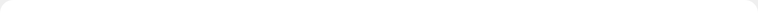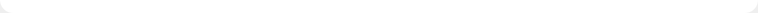# What is Sudoku?

## History

Sudoku is an easy to learn logic-based number placement puzzle. The word Sudoku is short for Su-ji wa dokushin ni kagiru which means "the numbers must be single".

The roots of the Sudoku puzzle are in the Switzerland. Leonhard Euler created "carré latin" in the 18h century which is similar to a Sudoku puzzle but without the additional constraint on the contents of individual regions. The first real Sudoku was published in 1979 and was invented by Howard Garns, an American architect.

The real world wide popularity started in Japan in 1986 after it was published and given the name Sudoku by Nikoli.

## Rules and TermsA Sudoku puzzle consists of 81 cells which are divided into nine columns, rows and regions. The task is now to place the numbers from 1 to 9 into the empty cells in such a way that in every row, column and 3×3 region each number appears only once.

A Sudoku has at least 17 given numbers but normally there are 22 to 30.

## Mathematics

A Sudoku is a logic-based and not a math-based puzzle. It is possible to make und solve a Sudoku puzzle with letters or even some symbols.

A little interesting point is that there are 6,670,903,752,021,072,936,960 possible Sudoku puzzles. So we can play umpteen Sudokus a day and there will still be new ones.

## How to solve a Sudoku (Example)

First of all, this is a guide for absolute beginners. I know there are better and/or more elegant ways to solve a Sudoku, but I only tried to write a little overview and not the ultimate Sudoku guide.

## Step 1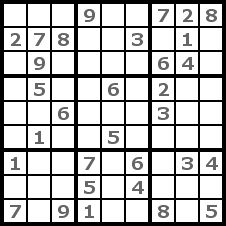Here in step 1 we have an example Sudoku. It has only the level easy but for this example it will be enough. A possible place to begin such a puzzle is to search for a number which appears most frequently.

## Step 2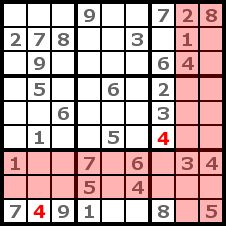We will try the 4 first. We look now for regions without a 4 and try to place the 4 by a process of elimination. For this purpose we identify which columns and rows contain a 4 because in the cells of these rows and columns we can't put the 4. If there is only one possible place left in a region, we place the 4 there. That's how we got the two 4s in the picture. This strategy is named cross-hatching.

## Step 3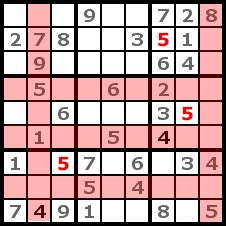We repeat the cross-hatching with the number 5. This is even more effective than with the number 4 because we can place three 5s.

## Step 4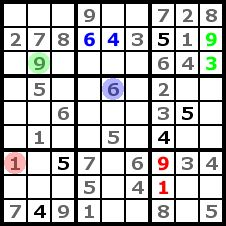In this step we can now complete the rows, columns and regions with only two empty cells left. This is easy to do because for one cell of each two cell groups there is only one possible number left. To make this easier to understand, I highlighted it with colors. Doing this now will make cross-hatching and counting simpler in the next steps.

## Step 5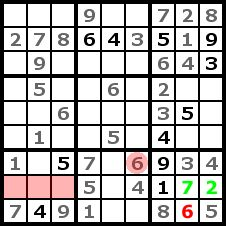At the lower left region we can't determine the exact position of the number 6, but we know into which row we have to put it (the three red cells). Therefore we can now place the 6 in the lower right region. Then we can get the 7 and 2 like in step 4.

## Step 6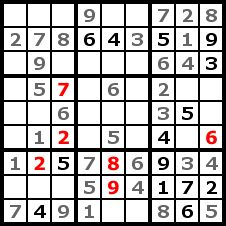Now we can't find any more numbers by cross-hatching. That's why we try it with counting. We count regions, rows, and columns to identify missing numerals for a cell. If we found a cell with only one possible number left after counting we place it into the cell. It is helpful to write little numbers into a cell to help memorize the findings of the counting. By counting, we got six numbers in this step.

## Step 7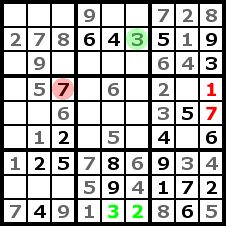In the same way as step 4 we can now complete a row and a column of our puzzle.

## Step 8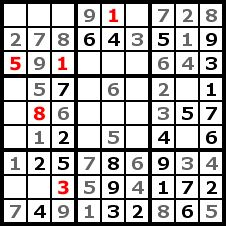We can find a few numbers in this step by counting like in step 6.

## Step 9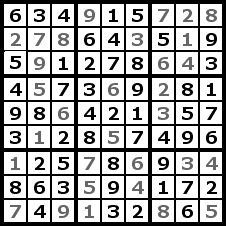And now we have the solutions to the Sudoku Puzzle. We skipped a few steps because there wasn't anything new in them but only repetitions of the other steps.